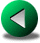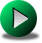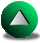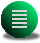Previous: Presentation of the Numerical
Next: Finite Difference Models
Up: Presentation of the Numerical

# The Time Discretization

Several time-stepping schemes are considered and used in conjunction with one of the spatial discretization techniques proposed in the following sections. For clarity, we review the time-stepping techniques separately in this section. Let us consider the equation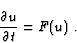(2.3)

The time-operator can be finite-differenced using a Taylor's series expansion truncated after the first term: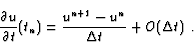(2.4)

The simplest time discretization consists then of integrating (2.3) given the previous time-step fields. This formulation corresponds to the so-called explicit forward Euler scheme and is only first order accurate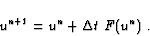(2.5)

This formulation is usually recommended for the integration of the dissipative or friction terms, because no large precision is required in time, as long as the small scale numerical noise are damped (and the scheme is stable). For ensuring stability, a condition on the magnitude of the time step,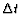, applies. For instance, when(a viscous dissipation term), this condition is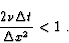(2.6)

Unfortunately, the forward Euler scheme is not neutral for various problems, in the sense that some quantities such as mass, momentum or energy are not conserved but may decay or grow as the simulation is advanced in time. When these quantities grow with time, the model is of course unstable. This happens, for instance, when F(u) represents the Coriolis terms. In order to better conserve certain quantities, a better scheme is the explicit leapfrog scheme(2.7)

based on a second order truncation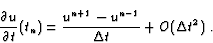(2.8)

The leapfrog scheme is thus centered in time. As the Euler scheme, this scheme is restricted to certain conditions for stability. For instance, if F(u)represents an advection or a wave propagation problem and using the definition that the Courant number is given by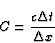(2.9)

where c is a phase speed or an advection velocity, the CFL (Courant-Friedrich-Levy) condition implies that

 C<1 (2.10)

for stability. The Leapfrog scheme is neutral and conditionally stable for problems involving, for instance, Coriolis or nonlinear advection terms, and is unstable for dissipative terms. Moreover, the leapfrog scheme requires a time-filtering, because the non-linearities and round-off errors lead to a decoupling of the solution between even and odd time steps. In order to avoid restriction of time-step magnitude, other time-integrations techniques were introduced. They include implicit and semi-implicit schemes. Nonetheless, these schemes have to respect a certain condition on the Courant number for ensuring a good accuracy. The semi-implicit 2.1 scheme consists of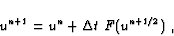(2.11)

where F(un+1/2) = 1/2 ( F(un+1) + F(un) ) and the fully-implicit scheme (also called the backward Euler scheme) is implemented as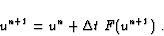(2.12)

The advantage of the semi-implicit treatment is that the time-operator is centered and second-order. For the Coriolis terms, the fully-implicit is dissipative, and the semi-implicit (centered in time) is neutral. For the treatment of the fast linear gravity waves present in the shallow water equations, the advantage of using a semi-implicit or fully-implicit technique is that there is no restriction on time-steps (the domain of stability of the models is extended) but at the expense of solving a matrix problem due to the coupling of the variables through partial derivatives. The disadvantage of these two techniques is that some physical processes, such as gravity waves, are slowed down if a too large time-step is used (i.e., C>1). This may have consequences for the interactions of important dynamical processes (the geostrophic and ageostrophic modes) and, therefore, this may lead to a less accurate representation of the cascade of energy (as mentioned in Bartello and Thomas, 1996).

The non-linear advective terms,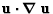, require a special treatment. When we consider the computation of, they can be computed using the previous time step as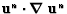. Then, if the time-operator is centered and leapfrog, the non-linear terms are neutrally treated, otherwise, they are off-centered for the other time-integration schemes and may be unstable or dissipative depending on the time integration techniques. The non-linear terms can be treated implicitly as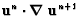or fully implicit using an iterative procedure. Another way is to use an explicit 4th order Adams-Bashforth formulation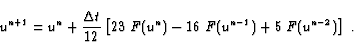(2.13)

The scheme is off-centered. It requires saving fields from several previous time-steps and the time-step is limited by a CFL condition. Use of Runge-Kutta techniques is also possible, the fourth order one having the advantage of good quadratic conserving properties, such as for the energy. But Runge-Kutta techniques require sub-step time integrations as in this 4th order example: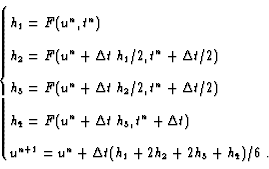(2.14)

The Runge-Kutta formulations are neutral for all phenomena, very accurate, and require a CFL condition. Adams-Bashforth formulations are usually recommended for non-linear integrations, but have the practical disadvantage of requiring smaller time-steps than equivalent order Runge-Kutta integrations, to the point that there is no definite advantage of one technique over the other2.2. Hereafter, we tend to use the 4th order Runge Kutta integration because of its accuracy and because it does not require any time filtering.

A completely different time-stepping approach consists of using the Lagrangian framework (the grid follows the particles) instead of the Eulerian framework implicitly assumed previously (the grid is fixed in time). The Lagrangian time-integration takes advantage of the fact that the dynamical equations are simplified when written in a Lagrangian form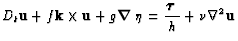(2.15)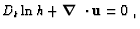(2.16)

where Dt is the Lagrangian or total time derivative. This is another way of saying that the particle trajectory is the characteristic line for the advective-only problem. Hence, the problematic non-linear terms appearing in the equations do not appear explicitly (except for the term in the mass balance). The main difficulty is in following the particles that form the flow, and especially expressing the right-hand-side terms. In order to avoid this problem, the so-called semi-Lagrangian formulation was developed which takes advantage of both the Lagrangian and Eulerian frameworks (see Staniforth and Côté, 1991, for a review). The right-hand-side terms are discretized on the Eulerian framework (in which derivatives are easy to express) and the time-derivative is treated on the Lagrangian framework. The advantage is in keeping a fixed grid or domain in time. An interpolation procedure is used in order to transfer information from the Eulerian grid to the Lagrangian grid (the particle trajectories). As the equations are time-stepped along the advective characteristic lines (the particle trajectories), there are no limitations imposed by numerical stability on the magnitude of the time-step due to the advective terms. Therefore, the method is effective in advection dominated flows. To be precise, according to Bartello and Thomas (1996), the method is effective only if the spectrum of energy is very steep (not too much energy at the smallest scales). Moreover, a semi-implicit or fully-implicit method can be added to the semi-Lagrangian treatment of the equations [Robert, 1981]. Thus the model has virtually no limitations due to stability regarding time-step magnitude with respect to any physical process described by the momentum equations. However, the presence of orography is troublesome in semi-Lagrangian methods, effectively reducing the allowable time-step [Ritchie and Tanguay, 1996]. The advantage of using semi-Lagrangian methods in an ocean where the topography is steep is, hence, unclear.

Since the equations are iterated in time, the interpolation can be very damaging to the conservation properties of the flow (mass or energy). That is why modelers have to use high order interpolation schemes (cubic or more). Nonetheless, the interpolation technique is usually responsible for a large numerical dissipation, difficult to minimize. On the other hand, these models can run without explicit eddy-viscosity or diffusivity. Proponents of the semi-Lagrangian method never fail to mention that their models run without explicit numerical viscosity, whereas opponents note that semi-Lagrangian models offer no control over this implicit viscosity. Another disadvantage of the semi-Lagrangian technique when coupled to the semi-implicit or implicit method is related to the same argument against the semi-implicit and implicit methods. Namely, that too large a time-step distorts the physical processes and misrepresents the real cascade of energy.Previous: Presentation of the Numerical
Next: Finite Difference Models
Up: Presentation of the Numerical

Frederic Dupont
2001-09-11